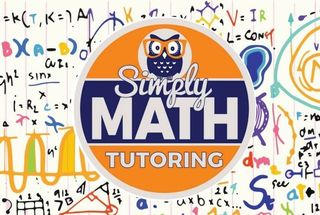\$849.00 - \$849.00

# 10th Grade – 4 week Math BootCamp• Ages:

Details of 4 week Camp:

→    Complete a table: quadratic equations
→    Solve a quadratic equation using square roots
→    Solve a quadratic equation using the zero product property
→    Solve a quadratic equation by factorising
→    Complete the square
→    Solve a quadratic equation by completing the square
→    Using the discriminant

2) lines and segments
→    Lines, line segments and half lines
→    Lengths of segments on number lines
→    Midpoints
→    Congruent line segments
→    Perpendicular Bisector Theorem
→    Midpoint formula
→    Distance formula

Circles
→    Parts of a circle
→    Central angles
→    Arc measure and arc length
→    Area of sectors
→    Circle measurements: mixed review
→    Arcs and chords
→    Tangent lines

Trigonometry
→    Trigonometric ratios: sin, cos and tan
→    Trigonometric ratios: csc, sec and cot
→    Trigonometric functions of complementary angles
→    Find trigonometric functions of special angles
→    Find trigonometric functions using a calculator
→    Inverses of trigonometric functions
→    Trigonometric ratios: find a side length

Linear equations
→    Identify linear equations
→    Find the slope of a graph
→    Find the slope from two points
→    Find a missing coordinate using slope
→    Slope-intercept form: find the slope and y-intercept
→    Slope-intercept form: graph an equation
→    Slope-intercept form: write an equation from a graph
→    Slope-intercept form: write an equation
→    Slope-intercept form: write an equation from a table
→    Slope-intercept form: write an equation from a word problem

Logic
→    Identify hypotheses and conclusions
→    Counterexamples
→    Truth tables
→    Truth values
→    Conditionals
→    Negations
→    Converses, inverses and contrapositives

Matrices
→    Matrix vocabulary
→    Matrix operation rules